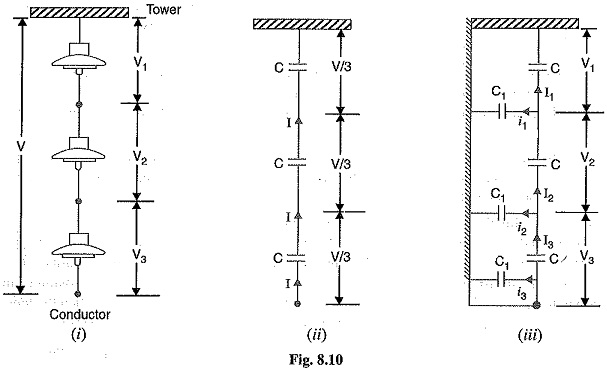## Potential Distribution Over Suspension Insulator String:

A string of suspension insulator consists of a number of porcelain discs connected in series through metallic links. Fig. 8.10 (i) shows 3-disc string of suspension insulator. The porcelain portion of each disc is in between two metal links. Therefore, each disc forms a capacitor C as shown in Fig. 8.10 (ii). This is known as mutual capacitance or self-capacitance. If there were mutual capacitance alone, then charging current would have been the same through all the discs and consequently voltage across each unit would have been the same i.e., V/3 as shown in Fig. 8.10 (ii). However, in actual practice, capacitance also exists between metal fitting of each disc and tower or earth. This is known as shunt capacitance C1. Due to shunt capacitance, charging current is not the same through all the discs of the string [See Fig. 8.10 (iii)]. Therefore, voltage across each disc will be different. Obviously, the disc nearest to the line conductor will have the maximum voltage. Thus referring to Fig. 8.10 (iii), V3 will be much more than V2 or V1.The following points may be noted regarding the potential distribution over a string of suspension insulators :

1.The voltage impressed on a string of suspension insulator does not distribute itself uniformly across the individual discs due to the presence of shunt capacitance.

2.The disc nearest to the conductor has maximum voltage across it. As we move towards the cross-arm, the voltage across each disc goes on decreasing.

3.The unit nearest to the conductor is under maximum electrical stress and is likely to be punctured. Therefore, means must be provided to equalize the potential across each unit.

4.If the voltage impressed across the string were d.c., then voltage across each unit would be the same.. It is because  insulator capacitance are ineffective for d.c.

Scroll to Top